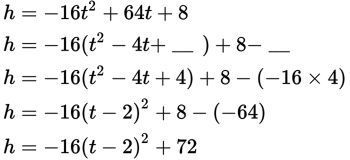# SAT Math Multiple Choice Question 360: Answer and Explanation

### Test Information

Question: 360

15. A projectile is any moving object that is thrown near the Earth's surface. The path of the projectile is called the trajectory and can be modeled by a quadratic equation, assuming the only force acting on the motion is gravity (no friction). If a projectile is launched from a platform 8 feet above the ground with an initial velocity of 64 feet per second, then its trajectory can be modeled by the equation h = –16t2 + 64t + 8, where h represents the height of the projectile t seconds after it was launched. Based on this model, what is the maximum height in feet that the projectile will reach?

• A. 72
• B. 80
• C. 92
• D. 108

Explanation:

A

Difficulty: Hard